On the Forward Invariance of Neural ODEs

## On the Forward Invariance of Neural ODEs

ICML 2023

Invariance of Neural ODEs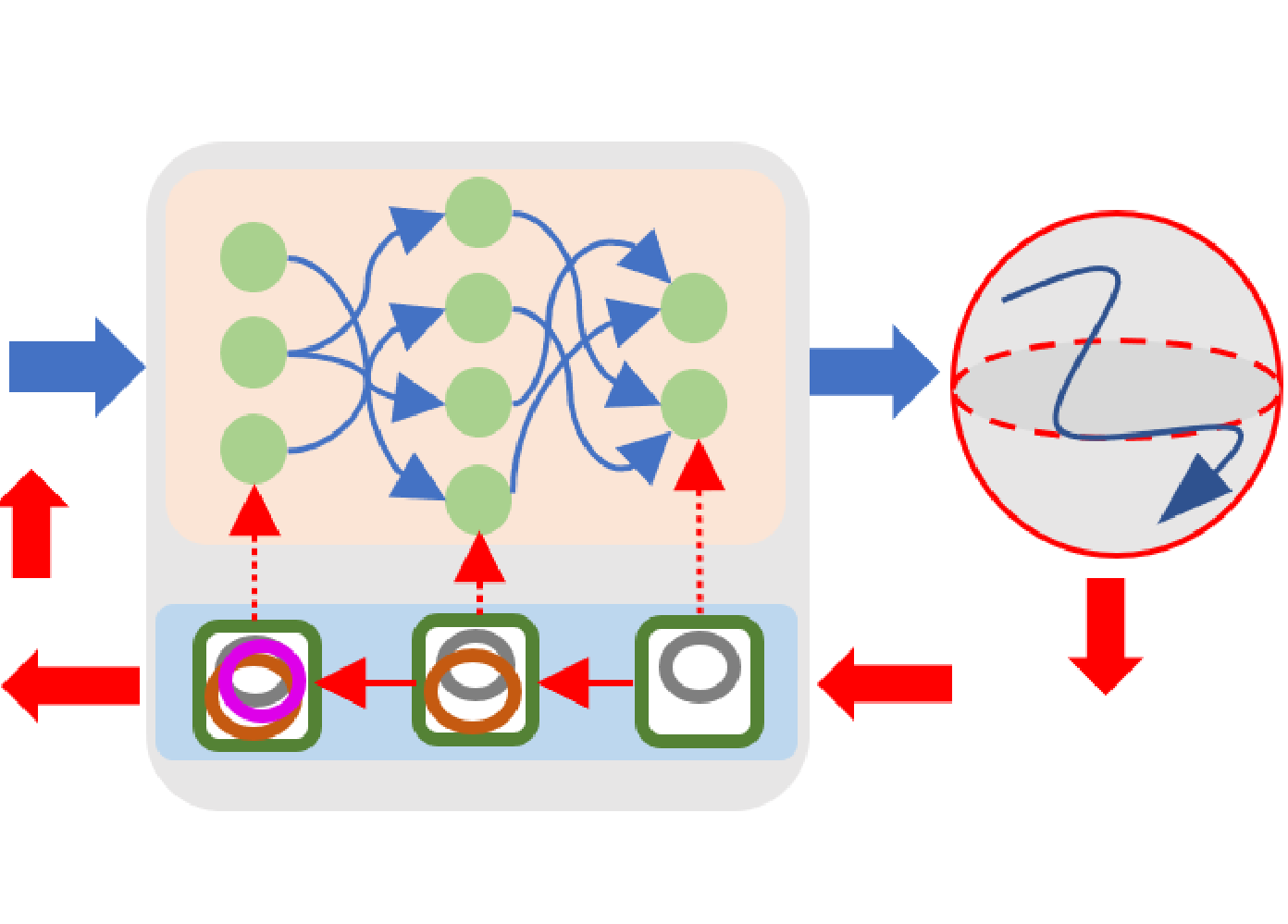Neural ODEs are continuous models that excel in learning representations from data with temporal properties, such as modeling system dynamics and controllers. Ensuring the output of neural ODEs to satisfy a certain performance (such as safety) is necessary for a variety of settings, which is defined as the forward invariance of neural ODEs. We propose a new method to ensure neural ODEs with invariance properties using Control Barrier Functions (CBFs).

What are CBFs? CBFs transform nonlinear optimizations to convex optimizations with provable guarantees.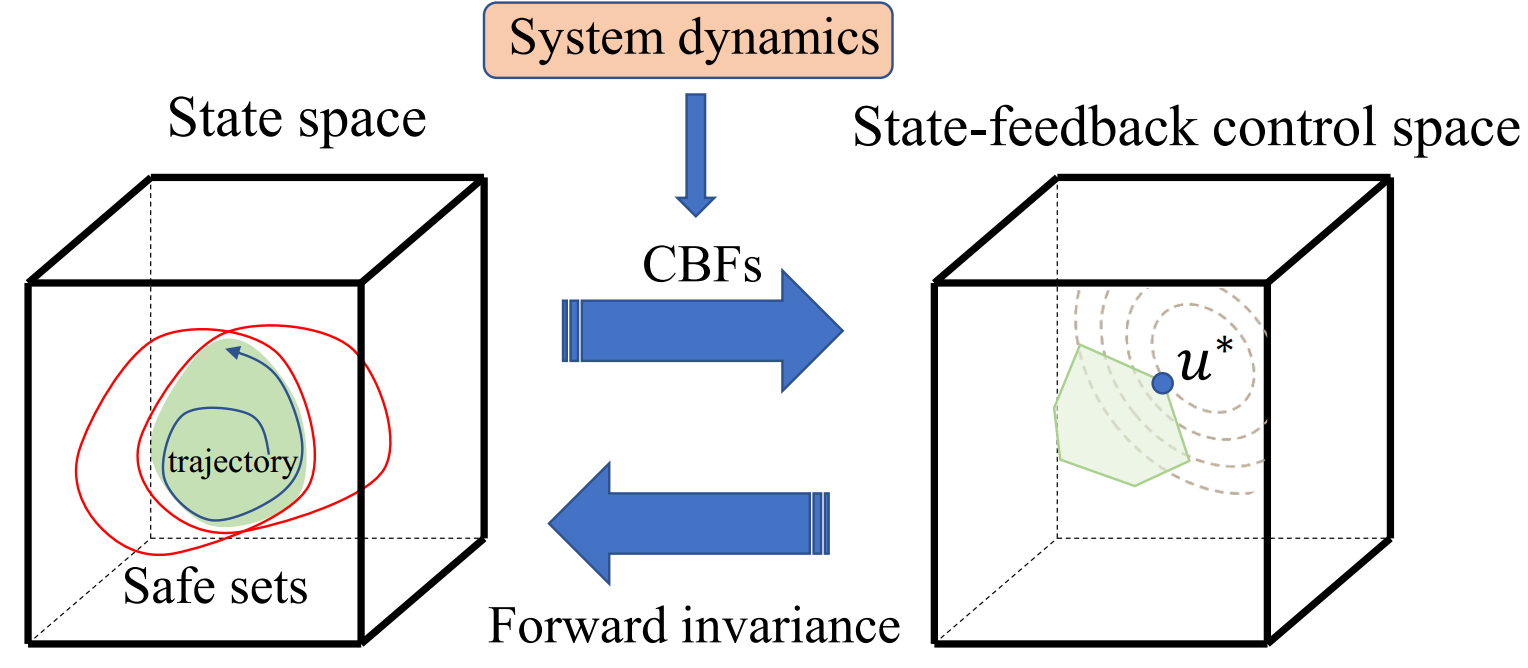Spiral Curve Regression with Specifications

The neural ODE is used to model a spiral curve that avoids critical areas (red): invariance propagated to model parameters.replay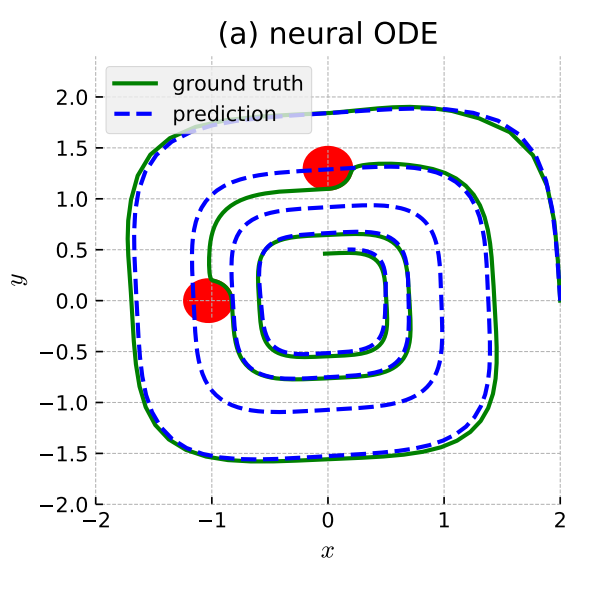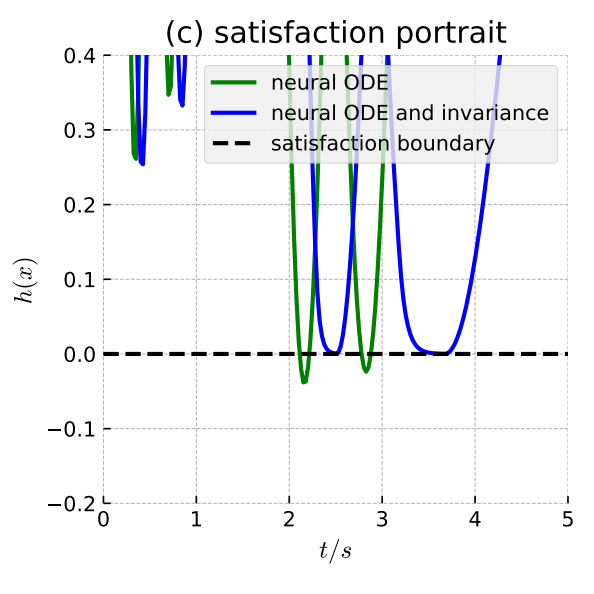Physical Dynamics Modeling

The neural ODE is used to model the dynamics of Walker2D in Gym: invariance propagated to model parameters.

Roof collision avoidance: left case without invariance, right case with invariance.replay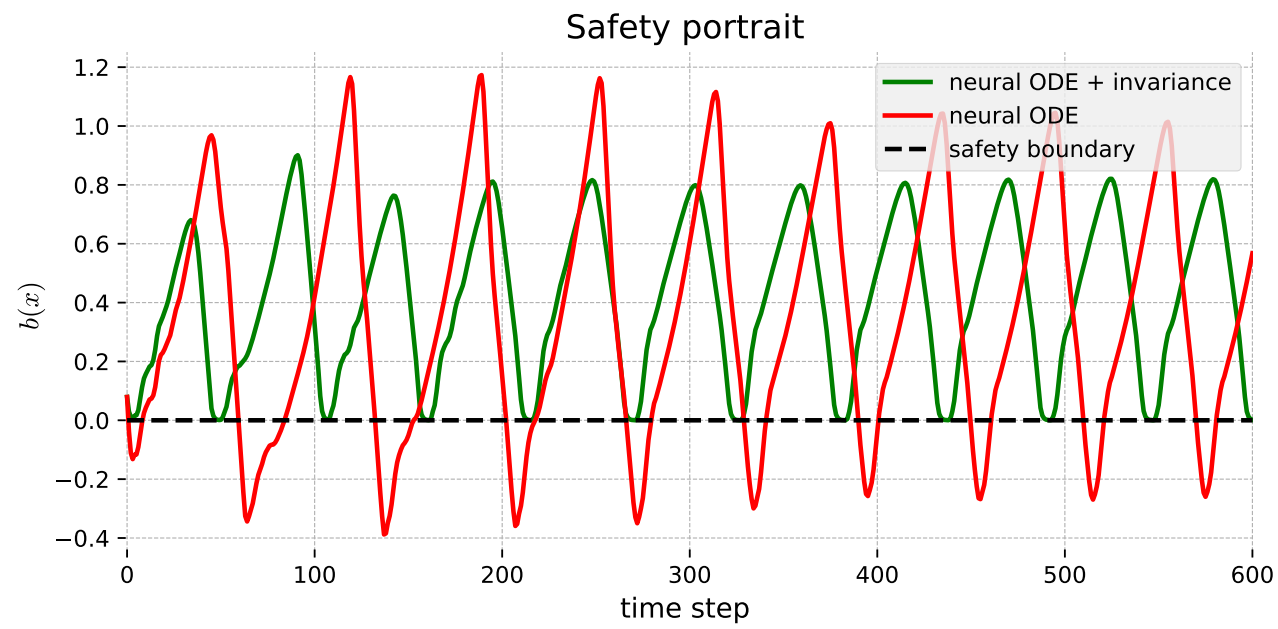Ball collision avoidance: left case without invariance, right case with invariance.replay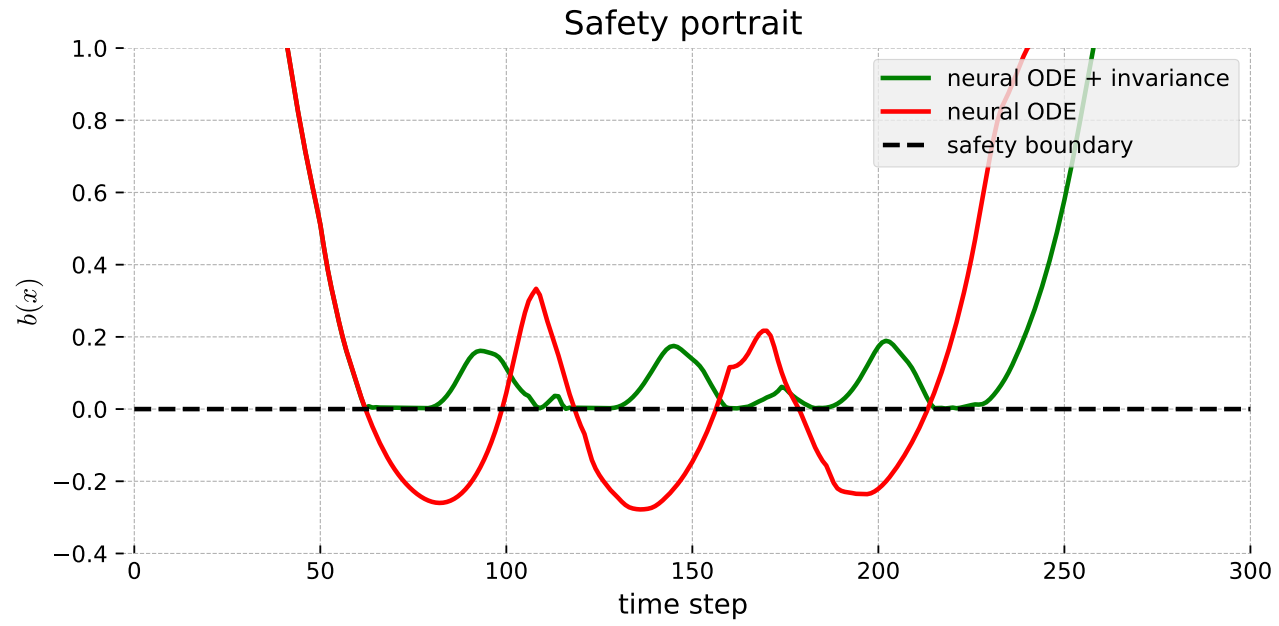Lidar-based Safe Driving

The neural ODE (with lidar as external input) is used to control an autonomous vehicle (i.e., a controller): invariance propagated to lidar (red trajectory-w/o invariance, green trajectory-w/ invariance, blue trajectory-conservative methods).

Original lidar-red; optimized lidar with invariance-green.replayOn the Forward Invariance of Neural ODEs
ICML 2023